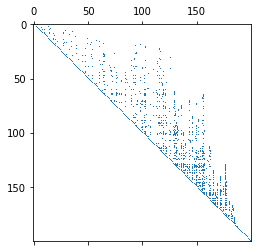# Sparse Matrix Factorizations and Fill-In¶

In :
import numpy as np
import scipy.linalg as la

import matplotlib.pyplot as pt

import random


Here's a helper routine to make a random symmetric sparse matrix:

In :
def make_random_sparse_matrix(n, row_fill):
nentries = (n*row_fill) // 2 # because of symmetry
data = np.random.randn(nentries)
rows = np.random.randint(0, n-1, nentries)
cols = np.random.randint(0, n-1, nentries)

import scipy.sparse as sps

coo = sps.coo_matrix((data, (rows, cols)), shape=(n,n))

# NOTE: Cuthill-McKee applies only to symmetric matrices!
return (100*np.eye(n) + np.array(coo.todense() +  coo.todense().T))


Next, we will take a look at that matrix from a "birds eye view". Every entry with absolute value greater that $10^{-10}$ will show up as a 'dot':

In :
prec = 1e-10

np.random.seed(15)
random.seed(15)

A = make_random_sparse_matrix(200, 3)
print("%d non-zeros" % len(np.where(np.abs(A)>prec)))
pt.figure()
pt.spy(A, marker=",", precision=prec)

794 non-zeros

Out:
<matplotlib.lines.Line2D at 0x11c693cd0>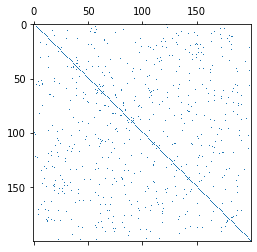Next, let's apply the same visualization to the inverse:

In :
Ainv = la.inv(A)
print("%d non-zeros" % len(np.where(np.abs(Ainv) > prec)))
pt.spy(Ainv, marker=",", precision=prec)

7148 non-zeros

Out:
<matplotlib.lines.Line2D at 0x10bfd9e50>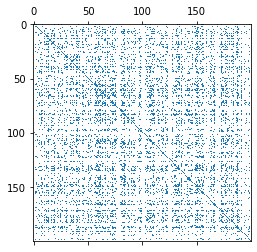And the Cholesky factorization:

In :
L = la.cholesky(A)
print("%d non-zeros" % len(np.where(np.abs(L) > prec)))
pt.spy(L, marker=",", precision=prec)

1819 non-zeros

Out:
<matplotlib.lines.Line2D at 0x11c6ec7d0>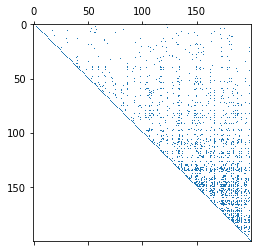Cholesky is often less bad, but in principle affected the same way.

## Reducing the fill-in¶

Define the degree of a row as the number of non-zeros in it.

In :
def degree(mat, row):
return len(np.where(mat[row]))

print(degree(A, 3))
print(degree(A, 4))
print(degree(A, 5))

2
4
3


Then find an ordering so that all the low degrees come first.

The Cuthill-McKee algorithm is a greedy algorithm to find such an ordering:

In :
def argmin2(iterable, return_value=False):
it = iter(iterable)
try:
current_argmin, current_min = next(it)
except StopIteration:
raise ValueError("argmin of empty iterable")

for arg, item in it:
if item < current_min:
current_argmin = arg
current_min = item

if return_value:
return current_argmin, current_min
else:
return current_argmin

def argmin(iterable):
return argmin2(enumerate(iterable))

In :
def cuthill_mckee(mat):
"""Return a Cuthill-McKee ordering for the given matrix.

See (for example)
Y. Saad, Iterative Methods for Sparse Linear System,
2nd edition, p. 76.
"""

# this list is called "old_numbers" because it maps a
# "new number to its "old number"
old_numbers = []
visited_nodes = set()
levelset = []

all_nodes = set(range(len(mat)))

while len(old_numbers) < len(mat):
if not levelset:
unvisited = list(all_nodes - visited_nodes)

if not unvisited:
break

start_node = unvisited[
argmin(degree(mat, node) for node in unvisited)]
old_numbers.append(start_node)
levelset = [start_node]

next_levelset = set()
levelset.sort(key=lambda row: degree(mat, row))
#print(levelset)

for node in levelset:
row = mat[node]
neighbors, = np.where(row)

for neighbor in neighbors:
if neighbor in visited_nodes:
continue

old_numbers.append(neighbor)

levelset = list(next_levelset)

return np.array(old_numbers, dtype=np.intp)

In :
cmk = cuthill_mckee(A)


Someone (empirically) observed that the reverse of the Cuthill-McKee ordering often does better than forward Cuthill-McKee.

So construct a permutation matrix corresponding to that:

In :
P = np.eye(len(A))[cmk[::-1]]


And then reorder both rows and columns according to that--a similarity transform:

In :
A_reordered = P @ A @ P.T

pt.spy(A_reordered, marker=",", precision=prec)

Out:
<matplotlib.lines.Line2D at 0x11daf4d10>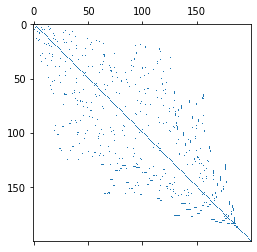Next, let's try Cholesky again:

In :
L = la.cholesky(A_reordered)
print("%d non-zeros" % len(np.where(np.abs(L) > prec)))
pt.spy(L, marker=",", precision=prec)

1188 non-zeros

Out:
<matplotlib.lines.Line2D at 0x11db93f10>